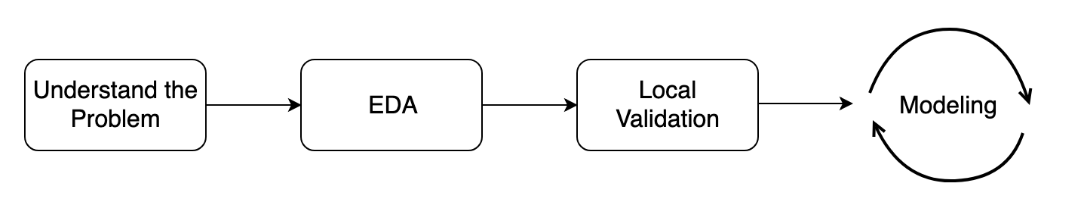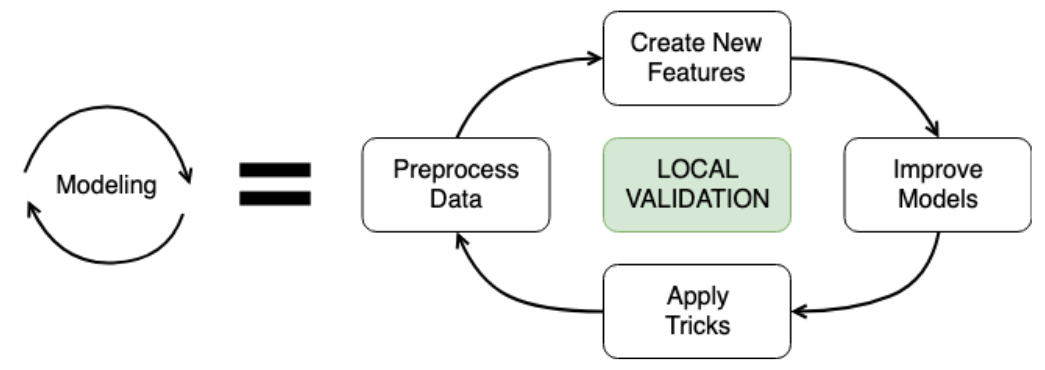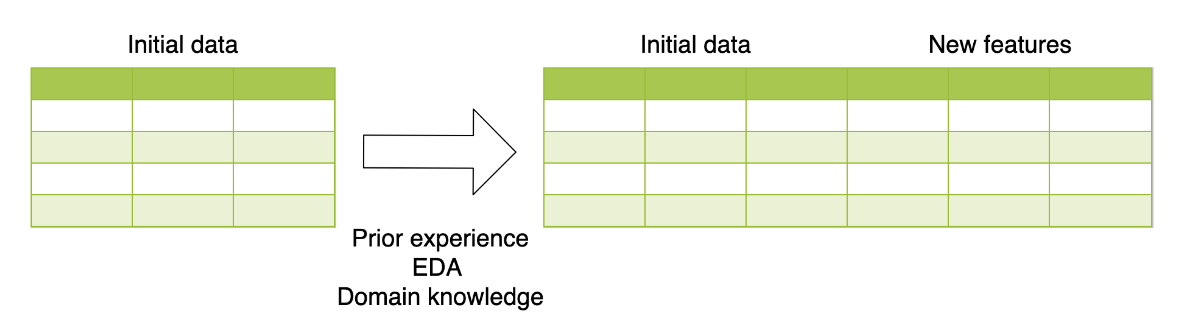import pandas as pd
import numpy as np
import matplotlib.pyplot as plt

plt.style.use('ggplot')
plt.rcParams['figure.figsize'] = (10, 8)


## Feature Engineering

• Solution workflow• Modeling Stage• Feature Engineering• Feature types
• Numerical
• Categorical
• Datetime
• Coordinates
• Text

### Arithmetical features

To practice creating new features, you will be working with a subsample from the Kaggle competition called "House Prices: Advanced Regression Techniques". The goal of this competition is to predict the price of the house based on its properties. It's a regression problem with Root Mean Squared Error as an evaluation metric.

Your goal is to create new features and determine whether they improve your validation score. To get the validation score from 5-fold cross-validation, you're given the get_kfold_rmse() function.

from sklearn.ensemble import RandomForestRegressor
from sklearn.model_selection import KFold
from sklearn.metrics import mean_squared_error

kf = KFold(n_splits=5, shuffle=True, random_state=123)

def get_kfold_rmse(train):
mse_scores = []

for train_index, test_index in kf.split(train):
train = train.fillna(0)
feats = [x for x in train.columns if x not in ['Id', 'SalePrice', 'RoofStyle', 'CentralAir']]

fold_train, fold_test = train.loc[train_index], train.loc[test_index]

# Fit the data and make predictions
# Create a Random Forest object
rf = RandomForestRegressor(n_estimators=10, min_samples_split=10, random_state=123)

# Train a model
rf.fit(X=fold_train[feats], y=fold_train['SalePrice'])

# Get predictions for the test set
pred = rf.predict(fold_test[feats])

fold_score = mean_squared_error(fold_test['SalePrice'], pred)
mse_scores.append(np.sqrt(fold_score))

return round(np.mean(mse_scores) + np.std(mse_scores), 2)

train = pd.read_csv('./dataset/house_prices_train.csv')

print('RMSE before feature engineering:', get_kfold_rmse(train))

# Find the total area of the house
train['totalArea'] = train['TotalBsmtSF'] + train['1stFlrSF'] + train['2ndFlrSF']

# Look at the updated RMSE
print('RMSE with total area:', get_kfold_rmse(train))

# Find the area of the garden
train['GardenArea'] = train['LotArea'] - train['1stFlrSF']
print('RMSE with garden area:', get_kfold_rmse(train))

# Find total number of bathrooms
train['TotalBath'] = train['FullBath'] + train['HalfBath']
print('RMSE with number of bathromms:', get_kfold_rmse(train))

RMSE before feature engineering: 36029.39
RMSE with total area: 35073.2
RMSE with garden area: 34413.55
RMSE with number of bathromms: 34506.78


You've created three new features. Here you see that house area improved the RMSE by almost $1,000. Adding garden area improved the RMSE by another $600. However, with the total number of bathrooms, the RMSE has increased. It means that you keep the new area features, but do not add "TotalBath" as a new feature.

### Date features

You've built some basic features using numerical variables. Now, it's time to create features based on date and time. You will practice on a subsample from the Taxi Fare Prediction Kaggle competition data. The data represents information about the taxi rides and the goal is to predict the price for each ride.

Your objective is to generate date features from the pickup datetime. Recall that it's better to create new features for train and test data simultaneously. After the features are created, split the data back into the train and test DataFrames. Here it's done using pandas' isin() method.

train = pd.read_csv('./dataset/taxi_train_chapter_4.csv')

taxi = pd.concat([train, test])

# Convert pickup date to datetime object
taxi['pickup_datetime'] = pd.to_datetime(taxi['pickup_datetime'])

# Create a day of week feature
taxi['dayofweek'] = taxi['pickup_datetime'].dt.dayofweek

# Create an hour feature
taxi['hour'] = taxi['pickup_datetime'].dt.hour

# Split back into train and test
new_train = taxi[taxi['id'].isin(train['id'])]
new_test = taxi[taxi['id'].isin(test['id'])]


## Categorical features

### Label encoding

Let's work on categorical variables encoding. You will again work with a subsample from the House Prices Kaggle competition.

Your objective is to encode categorical features "RoofStyle" and "CentralAir" using label encoding.

from sklearn.preprocessing import LabelEncoder

# Concatenate train and test together
houses = pd.concat([train, test])

# Label encoder
le = LabelEncoder()

# Create new features
houses['RoofStyle_enc'] = le.fit_transform(houses['RoofStyle'])
houses['CentralAir_enc'] = le.fit_transform(houses['CentralAir'])

# Look at new features

RoofStyle RoofStyle_enc CentralAir CentralAir_enc
0 Gable 1 Y 1
1 Gable 1 Y 1
2 Gable 1 Y 1
3 Gable 1 Y 1
4 Gable 1 Y 1

### One-Hot encoding

The problem with label encoding is that it implicitly assumes that there is a ranking dependency between the categories. So, let's change the encoding method for the features "RoofStyle" and "CentralAir" to one-hot encoding.

Recall that if you're dealing with binary features (categorical features with only two categories) it is suggested to apply label encoder only.

Your goal is to determine which of the mentioned features is not binary, and to apply one-hot encoding only to this one.

houses = pd.concat([train, test])

# Look at feature distributions
print(houses['RoofStyle'].value_counts(), '\n')
print(houses['CentralAir'].value_counts())

Gable      2310
Hip         551
Gambrel      22
Flat         20
Mansard      11
Shed          5
Name: RoofStyle, dtype: int64

Y    2723
N     196
Name: CentralAir, dtype: int64

le = LabelEncoder()
houses['CentralAir_enc'] = le.fit_transform(houses['CentralAir'])

# Create One-Hot encoded features
ohe = pd.get_dummies(houses['RoofStyle'], prefix='RoofStyle')

# Concatenate OHE features to houses
houses = pd.concat([houses, ohe], axis=1)

# Look at OHE features
houses[[col for col in houses.columns if 'RoofStyle' in col]].head(5)

RoofStyle RoofStyle_Flat RoofStyle_Gable RoofStyle_Gambrel RoofStyle_Hip RoofStyle_Mansard RoofStyle_Shed
0 Gable 0 1 0 0 0 0
1 Gable 0 1 0 0 0 0
2 Gable 0 1 0 0 0 0
3 Gable 0 1 0 0 0 0
4 Gable 0 1 0 0 0 0

## Target Encoding

• Mean target encoding
1. Calculate mean on the train, apply to the test
2. Split train into K folds, Calculate mean on (K-1) folds, apply to the K-th fold
3. Add mean target encoded feature to the model

### Mean target encoding

First of all, you will create a function that implements mean target encoding. Remember that you need to develop the two following steps:

1. Calculate the mean on the train, apply to the test
2. Split train into K folds. Calculate the out-of-fold mean for each fold, apply to this particular fold
def test_mean_target_encoding(train, test, target, categorical, alpha=5):
# Calculate global mean on the train data
global_mean = train[target].mean()

# Group by the categorical feature and calculate its properties
train_groups = train.groupby(categorical)
category_sum = train_groups[target].sum()
category_size = train_groups.size()

# Calculate smoothed mean target statistics
train_statistics = (category_sum + global_mean * alpha) / (category_size + alpha)

# Apply statistics to the test data and fill new categories
test_feature = test[categorical].map(train_statistics).fillna(global_mean)
return test_feature.values

def train_mean_target_encoding(train, target, categorical, alpha=5):
# Create 5-fold cross-validation
kf = KFold(n_splits=5,random_state=123, shuffle=True)
train_feature = pd.Series(index=train.index, dtype='float')

# For each folds split
for train_index, test_index in kf.split(train):
cv_train, cv_test = train.iloc[train_index], train.iloc[test_index]

# Calculate out-of-fold statistics and apply to cv_test
cv_test_feature = test_mean_target_encoding(cv_train, cv_test, target,
categorical, alpha)

# Save new feature for this particular fold
train_feature.iloc[test_index] = cv_test_feature
return train_feature.values

def mean_target_encoding(train, test, target, categorical, alpha=5):
# Get the train feature
train_feature = train_mean_target_encoding(train, target, categorical, alpha)

# Get the test feature
test_feature = test_mean_target_encoding(train, test, target, categorical, alpha)

# Return new features to add to the model
return train_feature, test_feature


### K-fold cross-validation

You will work with a binary classification problem on a subsample from Kaggle playground competition. The objective of this competition is to predict whether a famous basketball player Kobe Bryant scored a basket or missed a particular shot.

Train data is available in your workspace as bryant_shots DataFrame. It contains data on 10,000 shots with its properties and a target variable "shot\_made\_flag" -- whether shot was scored or not.

One of the features in the data is "game_id" -- a particular game where the shot was made. There are 541 distinct games. So, you deal with a high-cardinality categorical feature. Let's encode it using a target mean!

Suppose you're using 5-fold cross-validation and want to evaluate a mean target encoded feature on the local validation.

bryant_shots = pd.read_csv('./dataset/bryant_shots.csv')

# Create 5-fold cross-validation
kf = KFold(n_splits=5, random_state=123, shuffle=True)

# For each folds split
for train_index, test_index in kf.split(bryant_shots):
cv_train, cv_test = bryant_shots.iloc[train_index].copy(), bryant_shots.iloc[test_index].copy()

# Create mean target encoded feature
cv_train['game_id_enc'], cv_test['game_id_enc'] = mean_target_encoding(train=cv_train,
test=cv_test,
categorical='game_id',
alpha=5)

# Look at the encoding

      game_id  shot_made_flag  game_id_enc
118  20000108             1.0     0.444531
3249  20200471             0.0     0.562617
6048  20400930             0.0     0.276686
3199  20200425             0.0     0.485156
7808  20500988             0.0     0.392894


You could see different game encodings for each validation split in the output. The main conclusion you should make: while using local cross-validation, you need to repeat mean target encoding procedure inside each folds split separately.

### Beyond binary classification

Of course, binary classification is just a single special case. Target encoding could be applied to any target variable type:

• For binary classification usually mean target encoding is used
• For regression mean could be changed to median, quartiles, etc.
• For multi-class classification with N classes we create N features with target mean for each category in one vs. all fashion The mean_target_encoding() function you've created could be used for any target type specified above. Let's apply it for the regression problem on the example of House Prices Kaggle competition.

Your goal is to encode a categorical feature "RoofStyle" using mean target encoding.

train = pd.read_csv('./dataset/house_prices_train.csv')

# Create mean target encoded feature
train['RoofStyle_enc'], test['RoofStyle_enc'] = mean_target_encoding(train=train,
test=test,
target='SalePrice',
categorical='RoofStyle',
alpha=10)
# Look at the encoding
test[['RoofStyle', 'RoofStyle_enc']].drop_duplicates()

RoofStyle RoofStyle_enc
0 Gable 171565.947836
1 Hip 217594.645131
98 Gambrel 164152.950424
133 Flat 188703.563431
362 Mansard 180775.938759
1053 Shed 188267.663242

You observe that houses with the Hip roof are the most pricy, while houses with the Gambrel roof are the cheapest. It's exactly the goal of target encoding: you've encoded categorical feature in such a manner that there is now a correlation between category values and target variable. We're done with categorical encoders.

## Missing Data

• Impute missing data
• Numerical data
• Mean/median imputation
• Constant value imputation
• Categorical data
• Most frequent category imputation
• New category imputation

### Find missing data

Let's impute missing data on a real Kaggle dataset. For this purpose, you will be using a data subsample from the Kaggle "Two sigma connect: rental listing inquiries" competition.

Before proceeding with any imputing you need to know the number of missing values for each of the features. Moreover, if the feature has missing values, you should explore the type of this feature.

twosigma = pd.read_csv('./dataset/twosigma_rental_train_null.csv')

# find the number of missing values in each column
print(twosigma.isnull().sum())


id                 0
bathrooms          0
bedrooms           0
building_id       13
latitude           0
longitude          0
manager_id         0
price             32
interest_level     0
dtype: int64

building_id price
0 53a5b119ba8f7b61d4e010512e0dfc85 3000.0
1 c5c8a357cba207596b04d1afd1e4f130 5465.0
2 c3ba40552e2120b0acfc3cb5730bb2aa 2850.0
4 NaN 3350.0

### Impute missing data

You've found that "price" and "building_id" columns have missing values in the Rental Listing Inquiries dataset. So, before passing the data to the models you need to impute these values.

Numerical feature "price" will be encoded with a mean value of non-missing prices.

Imputing categorical feature "building_id" with the most frequent category is a bad idea, because it would mean that all the apartments with a missing "building_id" are located in the most popular building. The better idea is to impute it with a new category.

from sklearn.impute import SimpleImputer

# Create mean imputer
mean_imputer = SimpleImputer(strategy='mean')

# Price imputation
twosigma[['price']] = mean_imputer.fit_transform(twosigma[['price']])

# Create constant inputer
constant_imputer = SimpleImputer(strategy='constant', fill_value='MISSING')

# building_id imputation
twosigma[['building_id']] = constant_imputer.fit_transform(twosigma[['building_id']])

twosigma.isnull().sum()

id                0
bathrooms         0
bedrooms          0
building_id       0
latitude          0
longitude         0
manager_id        0
price             0
interest_level    0
dtype: int64